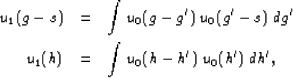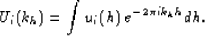Next: 2- and 3-D earth Up: A surface-related multiple prediction Previous: A surface-related multiple prediction

# One-dimensional earth and impulsive source

Let's define u0 as the primary wavefield and u1 the surface-related, first-order multiple wavefield recorded at the surface. If the earth varies only as a function of depth, then u will not depend on both s and g, but only on the offset, h=g-s. In this one-dimensional case, equation () becomes(144) (145)
where h'=g'-s. Equation () clearly represents a convolution, so can be computed by multiplication in the Fourier domain such that

 U1(kh) = U0(kh)2, (146)

where Ui(kh) is the Fourier transform of ui(h) defined by(147)

Equation () can be extended to deal with a smoothly varying earth by considering common shot-gathers (or common midpoint gathers) independently, and assuming the earth is locally one-dimensional in the vicinity of the shot, e.g., Rickett and Guitton (2000):

 U1(kh,s) = U0(kh,s)2. (148)

In practice, however, the primaries are not known and u0 is replaced by the data with primaries and multiples. A similar approach has been used by Kelamis and Verschuur (2000) for attenuating surface-related multiples on land data for relatively flat geology.Next: 2- and 3-D earth Up: A surface-related multiple prediction Previous: A surface-related multiple prediction
Stanford Exploration Project
5/5/2005